FreeBuf.COM网络安全行业门户，每日发布专业的安全资讯、技术剖析。FreeBuf+小程序

CTF现代密码
2020-11-17 14:52:43

## 前言：

1.素数

2.公约数与公倍数

3.欧拉函数

4.欧几里得算法

5.扩展欧几里得算法

6.同余

7.模运算

8.逆

9.中国剩余定理

10.逆元与同余式定理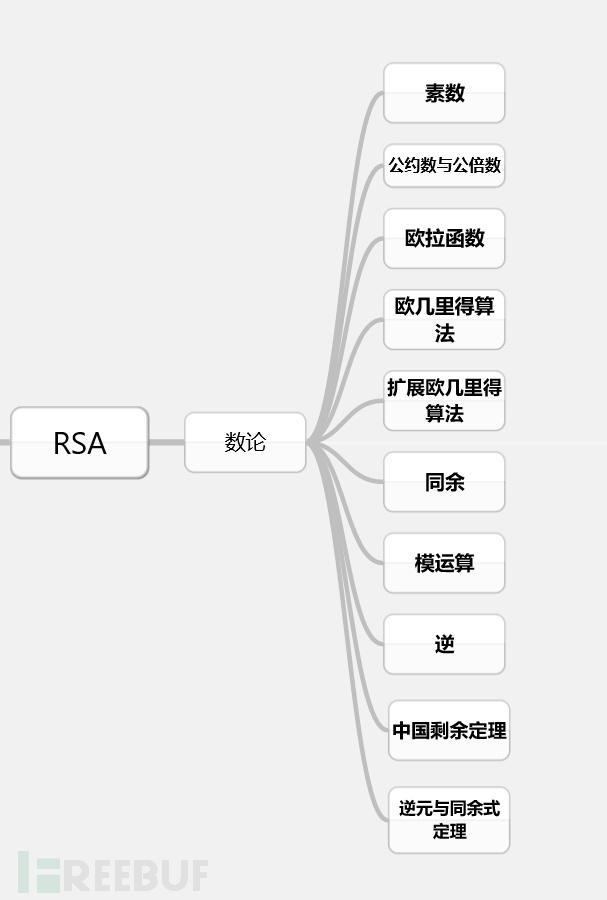## 1.素数：

3×4 = 12,不是素数。

11除了等于11×1以外，不能表示为其它任何两个整数的乘积，所以11是一个素数。

（1）如果p是素数，且p | ab（表示ab能被p整除），则p | a或 p | b ,即p 至少整除a与b中的一个。

（2）（算术基本定理）每个整数n ≥ 2 ,均可分解成素数幂之积：

n =

（3）素数有无穷多个。

## 3.欧拉函数

(1) 若 n 为一素数 P，则：φ(P) = P-1

(2) 如果P是素数，k≥则：φ() = (P-1)×

(3) 若 n 为任意两个互质的 数a,b的积，则：φ(a*b) = φ(a) × φ(b)

(4)对于整数n≥2，根据算术基本定理，n可以分解成唯一的形如 n=的分解式，则：φ(n)=n(1-)(1-)…(1-)

## 4.欧几里得(Euclid)算法

1.python代码实现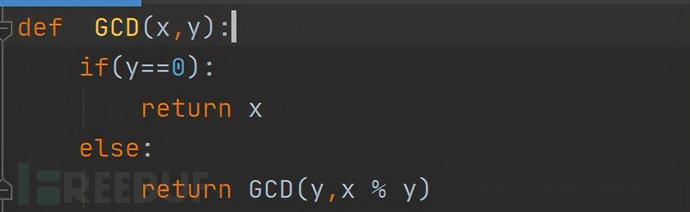2.python第三方库：

gmpy2.gcd(a,b) #求a,b的最大公约数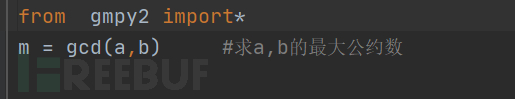Crypto.Util.number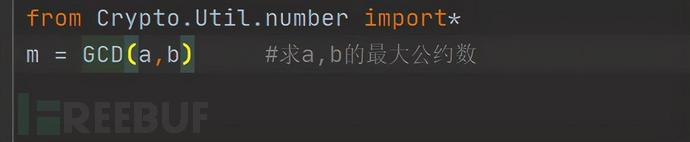## 5.扩展欧几里得算法

python代码实现:

gmpy2库函数gcdext()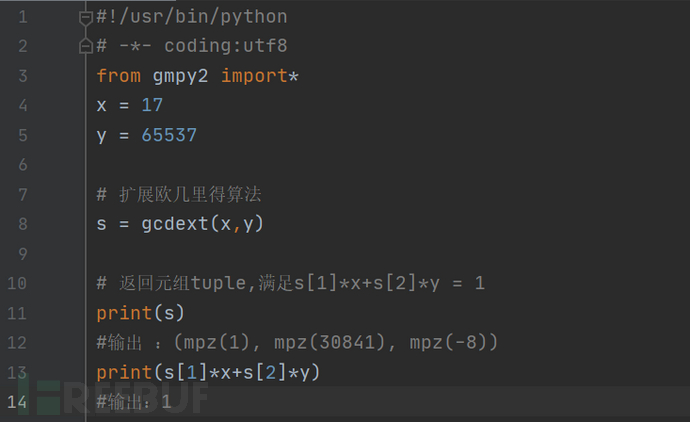## 6.同余

1. 自反性：a ≡ a (mod m)

2. 对称性：a ≡ b (mod m)，↔b ≡ c (mod m) ↔a ≡ c (mod m)

(1)若a ≡ b (mod m)，c ≡ d (mod m)

(2)若a ≡ b (mod m)，k>0，则ak ≡ bk (mod mk)

(3)若a ≡ b (mod m)，d是a，b的公因数，则 ≡

(4)若a ≡ b (mod m)，d|m，d>0，则: a ≡ b (mod d)

(5)若a ≡ b (mod m)，则:

(6)(a×b)mod m = (a mod m ×b mod m )mod m

(7)mod m = mod m

## 7.模运算

a模n的运算给出了a对模n的余数，这种运算称为模运算。注意：模运算的结果是从0到n-1的一个整数。

(a+b)mod m=((a mod m)+(b mod m)) mod m

(a-b)mod m=((a mod m)-(b mod m)) mod m

(a×b)mod m=((a mod m) ×(b mod m)) mod m

(a×(b+c))mod m=((a×b) mod m+(a×c) mod m) mod m

(a×a×a×a×a×a×a×a)mod n

ca≡1(mod m)

## 9.中国剩余定理

=(i=1,2,…,k),则同余方程组：## 10.逆元与同余式定理

1模运算重要公式：

(a+b) % m = (a % m + b % m) % m

(a-b) % m = (a % m - b % m) % m

(a*b) % m = (a % m * b % m) % m

% m = % m

2威尔逊定理： (wilson’s theorem)

3二次探测定理：

4费马小定理(Fermat)：

，推论:=a mod p

5欧拉定理(Euler)：

# CTF

+ 收入我的专辑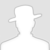• 0 文章数
• 0 评论数
• 0 关注者